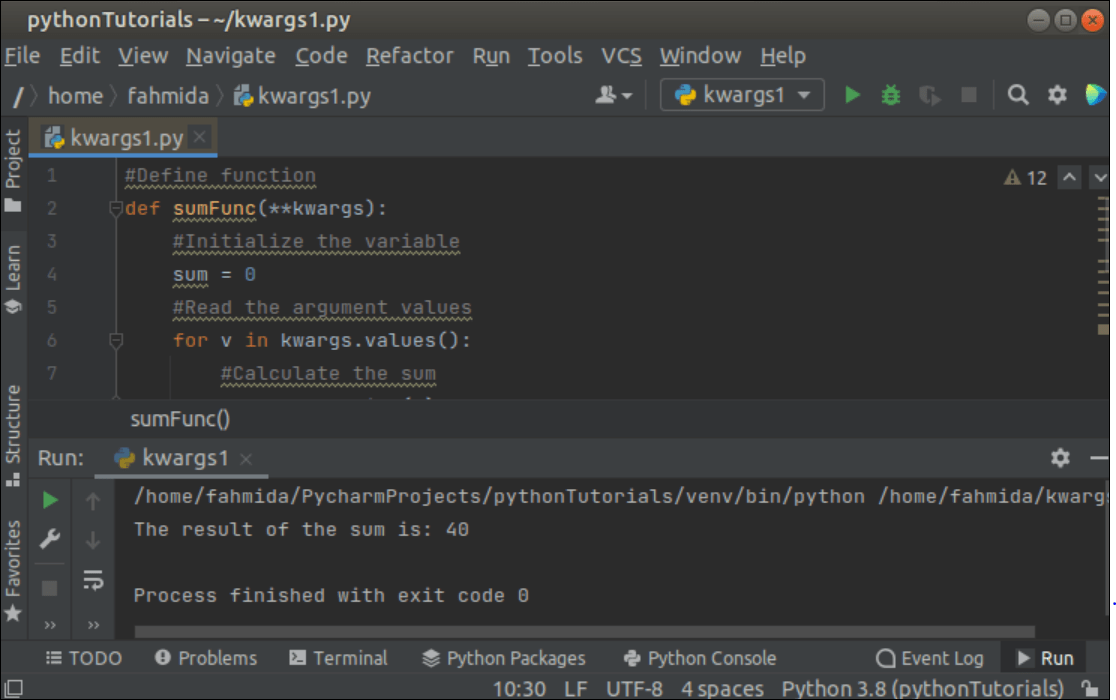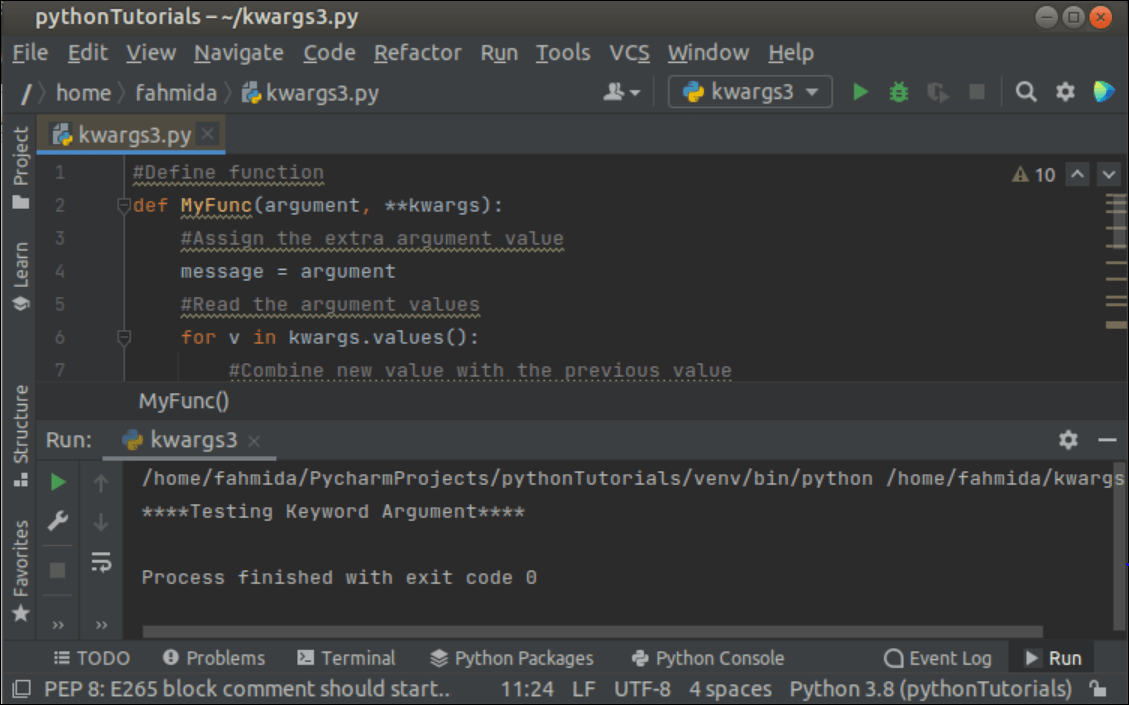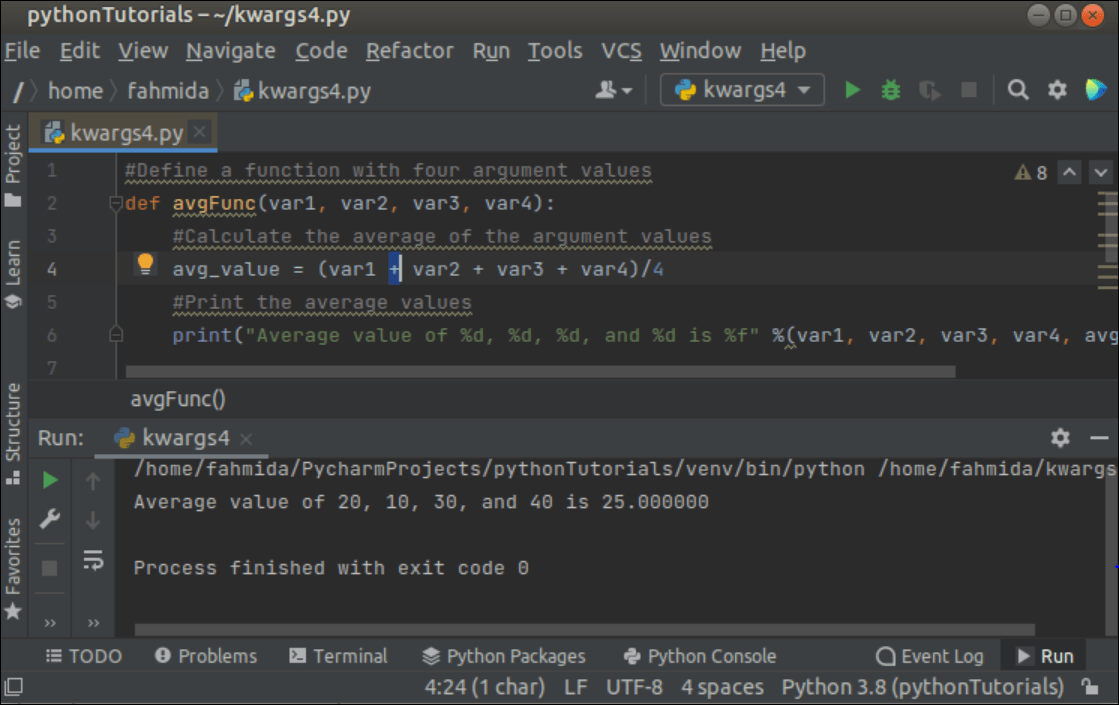Linux

# Use of Python kwargs

Two types of arguments can be passed to the Python function. These are *args (Non-keyword arguments) and **kwargs (Keyword arguments). The wildcard characters, ‘*’ and ‘**’ are used when the number of arguments is not defined. The **kwargs is used in the function to pass keyword-based variable-length arguments in the function. Different uses of kwargs in the Python function have been shown in this tutorial.

## Example-1: Read the Keyword Argument Values

Create a Python file with the following script that uses **kwargs as the argument in the function named sumFunc(). Two numbers have been passed as keyword arguments to the sumFunc() function. The kwargs.values() function has been used in the script to read only the argument values of the keyword argument by using the ‘for’ loop. The loop will iterate the argument values and print the sum of the values.

#Define function

def sumFunc(**kwargs):

#Initialize the variable

sum = 0

for v in kwargs.values():

#Calculate the sum

sum = sum + int(v)

#Print the sum value

print(“The result of the sum is: %d” %sum)

#Calling function with two arguments

sumFunc(num1=10, num2=30)

Output:

The following output will appear after executing the above script. The sum of 10 and 30 is 40, which has been printed in the output.## Example-2: Read the Keyword Argument Keys and Values

Create a Python file with the following script that uses **kwargs as the argument in the function named MyFunc(). Two strings have been passed as keyword arguments to the MyFunc() function. The kwargs.items() function has been used in the script to read the argument keys and values of the keyword argument by using the ‘for’ loop. The loop will iterate the key and value of the arguments and print the keys, values, and the concatenated string of the values.

#Define function

def MyFunc(**kwargs):

message =

#Read the argument names and value

for k, v in kwargs.items():

print(“%s = %s” % (k, v))

#Combine the string values

message = message + v

#Print the combined string values

print(message)

#Calling function with two arguments of string values

MyFunc(msg=‘Welcome to’, site=‘ LinuxHint’)

Output:

The following output will appear after executing the above script. The concatenated value of the argument values is, ‘Welcome to LinuxHint’. The keys with values and the concatenated string have been printed in the output.## Example-3: Use of kwargs with a Normal Argument

Create a Python file with the following script where the function takes the normal argument in the first argument, and the keyword argument in the second argument. The kwargs.values() function has been used in the script to read the argument values only from the keyword argument. Next, the values of the keyword argument have been concatenated with the value of the normal argument and printed later.

#Define function

def MyFunc(argument, **kwargs):

#Assign the extra argument value

message = argument

for v in kwargs.values():

#Combine new value with the previous value

message = message + v

#Add the extra argument value at the end of the variable

message = message + argument

#Print the variable

print(message)

#Calling function with two arguments of string values

MyFunc(‘****’, msg=‘Testing Keyword Argument’)

Output:

The following output will appear after executing the above script. The concatenated value of the normal argument and keyword argument is, ‘****Testing Keyword Argument****’ that is printed in the output.## Example-4: Pass the Keyword Argument by Using a Dictionary

Create a Python file with the following script where the function takes four normal arguments and print the average value of the argument values. Here, the kwargs variables has been declared as a dictionary object where the keys are the same as the normal argument variable names. Next, the function has been called by **kwargs.

#Define a function with four argument values

def avgFunc(var1, var2, var3, var4):

#Calculate the average of the argument values

avg_value = (var1 + var2 + var3 + var4)/4

#Print the average values

print(“Average value of %d, %d, %d, and %d is %f” %(var1, var2, var3, var4, avg_value))

#Declare a dictionary of four items

kwargs = {“var1”: 20, “var2”: 10, “var3”: 30, “var4”: 40}

#Call the function with the keyword arguments

avgFunc(**kwargs)

Output:

The following output will appear after executing the above script. The average value of 20, 10, 30, and 40 is 25 which has been printed in the output.## Example-5: Use of kwargs, Normal Argument, and Argument with the Default Value

Create a Python file with the following script where the function takes two normal arguments, two arguments with default values, and the keyword argument. The value of the 1st argument that is passed at the time of the function call, will be printed in the first output. The sum of the 1st, 2nd, and 3rd arguments will be printed in the second output. The value of the 4th argument will be printed in the third output. The value of the keyword argument will be printed as a dictionary in the fourth output.

”’

Define the function with undefined arguments,

defined arguments and keyword arguments

”’

def MyFunc(var1, var2, var3=20, var4=False, **kwargs):

#Print the first argument value

print(“The 1st argument value is “, var1)

#Print the sum of three argument values

print(“The sum of three argument values is “, var1 + var2 + var3)

#Print the 4th argument value

print(“The 4th argument value is “, var4)

#Print the keyword arguments

print(“The values of keyword arguments are :”, kwargs)

#Call the function

MyFunc(45, 35.89, arg1=11, arg2=22, arg3=66)

Output:

The following output will appear after executing the above script.## Conclusion

A function can be called with a different number of arguments at different times by using kwargs based on programming purposes. The uses of kwargs in the Python function have been explained in this tutorial properly for helping the Python users to use it in their script when required.